Trang chủ‎ > ‎IT‎ > ‎Data Science - Python‎ > ‎Tensorflow‎ > ‎

TensorFlow in a Nutshell — Part Three: All the Models

The fast and easy guide to the most popular Deep Learning framework in the world.

Make sure to check out the other articles here.

Overview

In this installment we will be going over all the abstracted models that are currently available in TensorFlow and describe use cases for that particular model as well as simple sample code. Full sources of working examples are in the TensorFlow In a Nutshell repo.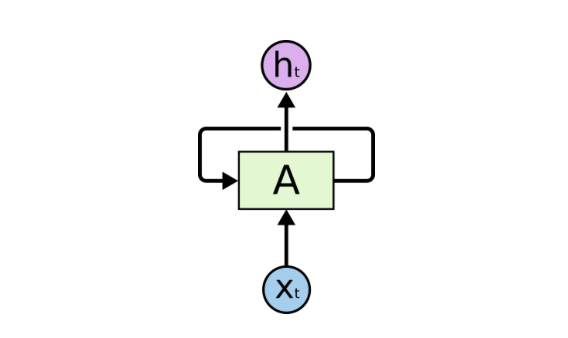A recurrent neural network

Recurrent Neural Networks

Use Cases: Language Modeling, Machine translation, Word embedding, Text processing.

Since the advent of Long Short Term Memory and Gated Recurrent Units, Recurrent Neural Networks have made leaps and bounds above other models in natural language processing. They can be fed vectors representing characters and be trained to generate new sentences based on the training set. The merit in this model is that it keeps the context of the sentence and derives meaning that “cat sat on the mat” means the cat is on the mat. Since the creation of TensorFlow writing these networks have become increasingly simpler. There are even hidden features covered by Denny Britz here that make writing RNN’s even simpler heres a quick example.

import tensorflow as tf
import numpy as np
# Create input data
X = np.random.randn(2, 10, 8)

# The second example is of length 6
X[1,6,:] = 0
X_lengths = [10, 6]

cell = tf.nn.rnn_cell.LSTMCell(num_units=64, state_is_tuple=True)
cell = tf.nn.rnn_cell.DropoutWrapper(cell=cell, output_keep_prob=0.5)
cell = tf.nn.rnn_cell.MultiRNNCell(cells=[cell] * 4, state_is_tuple=True)

outputs, last_states = tf.nn.dynamic_rnn(
cell=cell,
dtype=tf.float64,
sequence_length=X_lengths,
inputs=X)

result = tf.contrib.learn.run_n(
{"outputs": outputs, "last_states": last_states},
n=1,
feed_dict=None)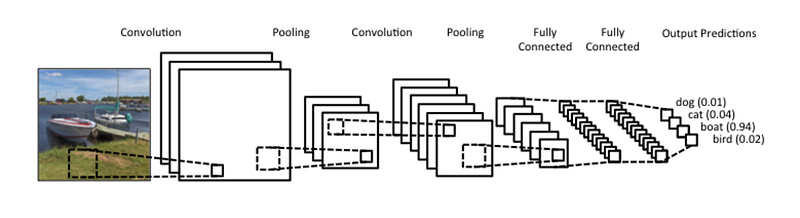Convolution Neural Network

Convolution Neural Networks

Use Cases: Image processing, Facial recognition, Computer Vision

Convolution Neural Networks are unique because they’re created in mind that the input will be an image. CNNs perform a sliding window function to a matrix. The window is called a kernel and it slides across the image creating a convolved feature.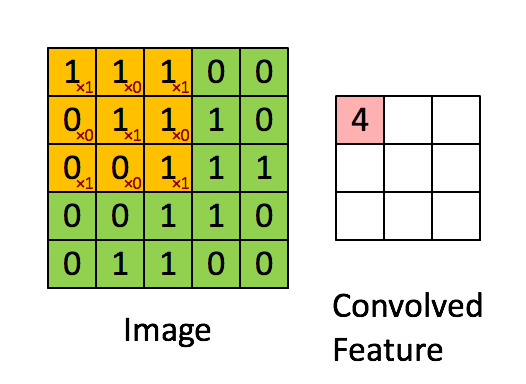from http://deeplearning.standford.edu/wiki/index.php/Feature_extraction_using_convolution

Creating a convolved feature allows for edge detection which then allows for a network to depict objects from pictures.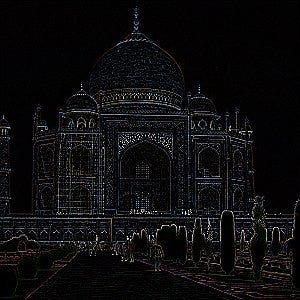edge detection from GIMP manual

The convolved feature to create this looks like this matrix below: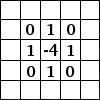Convolved feature from GIMP manual

Here’s a sample of code to identify handwritten digits from the MNIST dataset.

### Convolutional network
def max_pool_2x2(tensor_in):
return tf.nn.max_pool(
tensor_in, ksize=[1, 2, 2, 1], strides=[1, 2, 2, 1], padding='SAME')
def conv_model(X, y):
# reshape X to 4d tensor with 2nd and 3rd dimensions being image width and
# height final dimension being the number of color channels.
X = tf.reshape(X, [-1, 28, 28, 1])
# first conv layer will compute 32 features for each 5x5 patch
with tf.variable_scope('conv_layer1'):
h_conv1 = learn.ops.conv2d(X, n_filters=32, filter_shape=[5, 5],
bias=True, activation=tf.nn.relu)
h_pool1 = max_pool_2x2(h_conv1)
# second conv layer will compute 64 features for each 5x5 patch.
with tf.variable_scope('conv_layer2'):
h_conv2 = learn.ops.conv2d(h_pool1, n_filters=64, filter_shape=[5, 5],
bias=True, activation=tf.nn.relu)
h_pool2 = max_pool_2x2(h_conv2)
# reshape tensor into a batch of vectors
h_pool2_flat = tf.reshape(h_pool2, [-1, 7 * 7 * 64])
# densely connected layer with 1024 neurons.
h_fc1 = learn.ops.dnn(
h_pool2_flat, , activation=tf.nn.relu, dropout=0.5)
return learn.models.logistic_regression(h_fc1, y)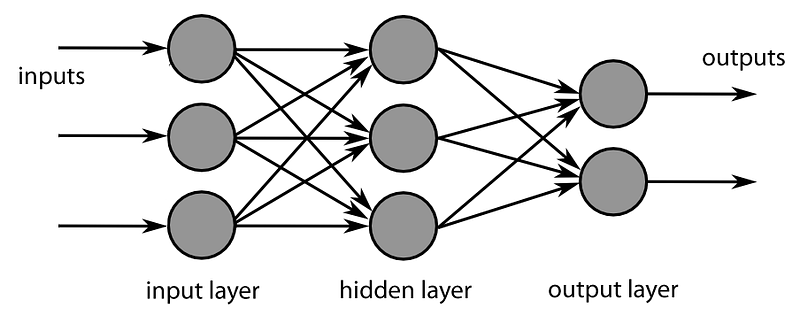Feed Forward Neural Networks

Use Cases: Classification and Regression

These networks consist of perceptrons in layers that take inputs that pass information on to the next layer. The last layer in the network produces the output. There is no connection between each node in a given layer. The layer that has no original input and no final output is called the hidden layer.

The goal of this network is similar to other supervised neural networks using back propagation, to make inputs have the desired trained outputs. These are some of the simplest effective neural networks for classification and regression problems. We will show how easy it is to create a feed forward network to classify handwritten digits:

def init_weights(shape):
return tf.Variable(tf.random_normal(shape, stddev=0.01))
def model(X, w_h, w_o):
h = tf.nn.sigmoid(tf.matmul(X, w_h)) # this is a basic mlp, think 2 stacked logistic regressions
return tf.matmul(h, w_o) # note that we dont take the softmax at the end because our cost fn does that for us
mnist = input_data.read_data_sets("MNIST_data/", one_hot=True)
trX, trY, teX, teY = mnist.train.images, mnist.train.labels, mnist.test.images, mnist.test.labels
X = tf.placeholder("float", [None, 784])
Y = tf.placeholder("float", [None, 10])
w_h = init_weights([784, 625]) # create symbolic variables
w_o = init_weights([625, 10])
py_x = model(X, w_h, w_o)
cost = tf.reduce_mean(tf.nn.softmax_cross_entropy_with_logits(py_x, Y)) # compute costs
train_op = tf.train.GradientDescentOptimizer(0.05).minimize(cost) # construct an optimizer
predict_op = tf.argmax(py_x, 1)
# Launch the graph in a session
with tf.Session() as sess:
# you need to initialize all variables
tf.initialize_all_variables().run()
for i in range(100):
for start, end in zip(range(0, len(trX), 128), range(128, len(trX)+1, 128)):
sess.run(train_op, feed_dict={X: trX[start:end], Y: trY[start:end]})
print(i, np.mean(np.argmax(teY, axis=1) ==
sess.run(predict_op, feed_dict={X: teX, Y: teY})))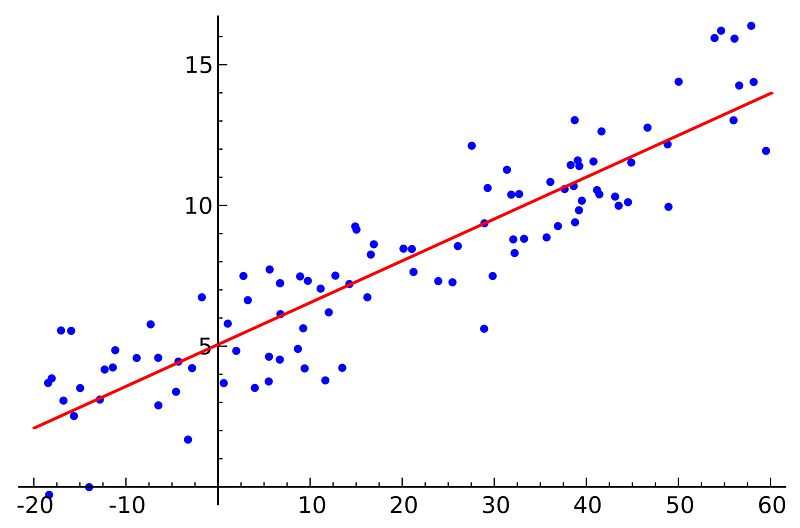Linear Models

Use Cases: Classification and Regression

Linear models take X values and produce a line of best fit used for classification and regression of Y values. For example if you have a list of house sizes and their price in a neighborhood you can predict the price of house given the size using a linear model.

One thing to note is that linear models can be used for multiple X features. For example in the housing example we can create a linear model given house sizes, how many rooms, how many bathrooms and price and predict price given a house with size, # of rooms, # of bathrooms.

import numpy as np
import tensorflow as tf
def weight_variable(shape):
initial = tf.truncated_normal(shape, stddev=1)
return tf.Variable(initial)
# dataset
xx = np.random.randint(0,1000,[1000,3])/1000.
yy = xx[:,0] * 2 + xx[:,1] * 1.4 + xx[:,2] * 3
# model
x = tf.placeholder(tf.float32, shape=[None, 3])
y_ = tf.placeholder(tf.float32, shape=[None])
W1 = weight_variable([3, 1])
y = tf.matmul(x, W1)
# training and cost function
cost_function = tf.reduce_mean(tf.square(tf.squeeze(y) - y_))
# create a session
sess = tf.Session()
# train
sess.run(tf.initialize_all_variables())
for i in range(10000):
sess.run(train_function, feed_dict={x:xx, y_:yy})
if i % 1000 == 0:
print(sess.run(cost_function, feed_dict={x:xx, y_:yy}))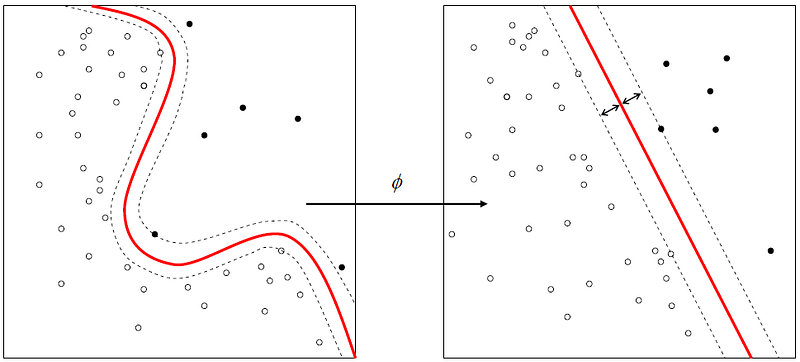Support Vector Machines

Use Cases: Currently only Binary Classification

The general idea behind a SVM is that there is an optimal hyperplane for linearly separable patterns. For data that is not linearly separable we can use a kernel function to transform the original data into a new space. SVMs maximize the margin around separating the hyperplane. They work extremely well in high dimensional spaces and and are still effective if the dimensions are greater than the number of samples.

def input_fn():
return {
'example_id': tf.constant(['1', '2', '3']),
'price': tf.constant([[0.6], [0.8], [0.3]]),
'sq_footage': tf.constant([[900.0], [700.0], [600.0]]),
'country': tf.SparseTensor(
values=['IT', 'US', 'GB'],
indices=[[0, 0], [1, 3], [2, 1]],
shape=[3, 5]),
'weights': tf.constant([[3.0], [1.0], [1.0]])
}, tf.constant([, , ])
price = tf.contrib.layers.real_valued_column('price')
sq_footage_bucket = tf.contrib.layers.bucketized_column(
tf.contrib.layers.real_valued_column('sq_footage'),
boundaries=[650.0, 800.0])
country = tf.contrib.layers.sparse_column_with_hash_bucket(
'country', hash_bucket_size=5)
sq_footage_country = tf.contrib.layers.crossed_column(
[sq_footage_bucket, country], hash_bucket_size=10)
svm_classifier = tf.contrib.learn.SVM(
feature_columns=[price, sq_footage_bucket, country, sq_footage_country],
example_id_column='example_id',
weight_column_name='weights',
l1_regularization=0.1,
l2_regularization=1.0)
svm_classifier.fit(input_fn=input_fn, steps=30)
accuracy = svm_classifier.evaluate(input_fn=input_fn, steps=1)['accuracy']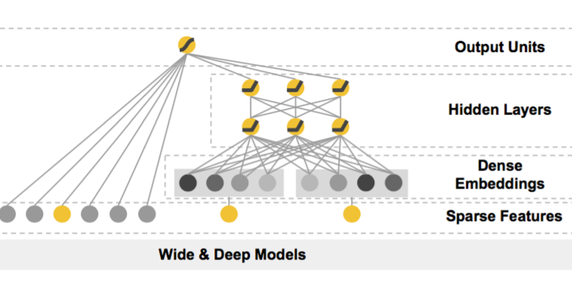Deep and Wide Models

Use Cases: Recommendation systems, Classification and Regression

Deep and Wide models were covered with greater detail in part two, so we won’t get too heavy here. A Wide and Deep Network combines a linear model with a feed forward neural net so that our predictions will have memorization and generalization. This type of model can be used for classification and regression problems. This allows for less feature engineering with relatively accurate predictions. Thus, getting the best of both worlds. Here’s a code snippet from part two’s github.

def input_fn(df, train=False):
"""Input builder function."""
# Creates a dictionary mapping from each continuous feature column name (k) to
# the values of that column stored in a constant Tensor.
continuous_cols = {k: tf.constant(df[k].values) for k in CONTINUOUS_COLUMNS}
# Creates a dictionary mapping from each categorical feature column name (k)
# to the values of that column stored in a tf.SparseTensor.
categorical_cols = {k: tf.SparseTensor(
indices=[[i, 0] for i in range(df[k].size)],
values=df[k].values,
shape=[df[k].size, 1])
for k in CATEGORICAL_COLUMNS}
# Merges the two dictionaries into one.
feature_cols = dict(continuous_cols)
feature_cols.update(categorical_cols)
# Converts the label column into a constant Tensor.
if train:
label = tf.constant(df[SURVIVED_COLUMN].values)
# Returns the feature columns and the label.
return feature_cols, label
else:
return feature_cols
m = build_estimator(model_dir)
m.fit(input_fn=lambda: input_fn(df_train, True), steps=200)
print m.predict(input_fn=lambda: input_fn(df_test))
results = m.evaluate(input_fn=lambda: input_fn(df_train, True), steps=1)
for key in sorted(results):
print("%s: %s" % (key, results[key]))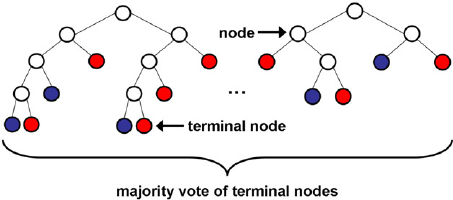Random Forest

Use Cases: Classification and Regression

Random Forest model takes many different classification trees and each tree votes for that class. The forest chooses the classification having the most votes.

Random Forests do not overfit, you can run as many treees as you want and it is relatively fast. Give it a try on the iris data with this snippet below:

hparams = tf.contrib.tensor_forest.python.tensor_forest.ForestHParams(
num_trees=3, max_nodes=1000, num_classes=3, num_features=4)
classifier = tf.contrib.learn.TensorForestEstimator(hparams)
data = iris.data.astype(np.float32)
target = iris.target.astype(np.float32)
monitors = [tf.contrib.learn.TensorForestLossMonitor(10, 10)]
classifier.fit(x=data, y=target, steps=100, monitors=monitors)
classifier.evaluate(x=data, y=target, steps=10)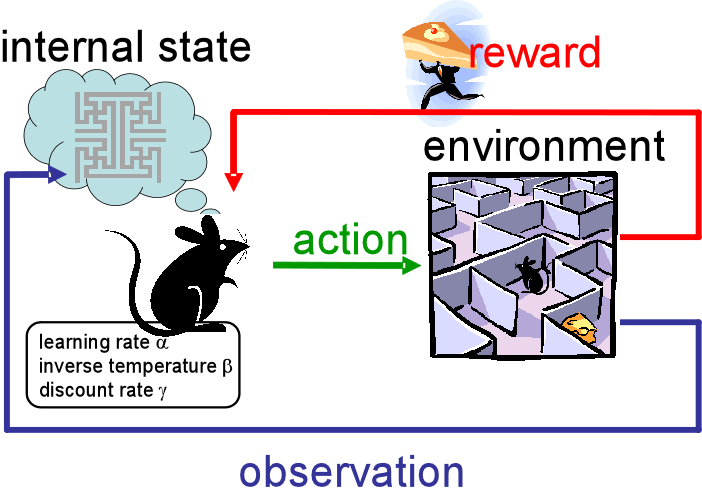Bayesian Reinforcement Learning

Use Cases: Classification and Regression

In the contrib folder of TensorFlow there is a library called BayesFlow. BayesFlow has no documentation except for an example of the REINFORCE algorithm. This algorithm is proposed in a paper by Ronald Williams.

REward Increment = Nonnegative Factor * Offset Reinforcement * Characteristic Eligibility

This network trying to solve an immediate reinforcement learning task, adjusts the weights after getting the reinforcement value at each trial. At the end of each trial each weight is incremented by a learning rate factor multiplied by the reinforcement value minus the baseline multiplied by characteristic eligibility. Williams paper also discusses the use of back propagation to train the REINFORCE network.

"""Build the Split-Apply-Merge Model.
Route each value of input [-1, -1, 1, 1] through one of the
functions, plus_1, minus_1. The decision for routing is made by
4 Bernoulli R.V.s whose parameters are determined by a neural network
applied to the input. REINFORCE is used to update the NN parameters.
Returns:
The 3-tuple (route_selection, routing_loss, final_loss), where:
- route_selection is an int 4-vector
- routing_loss is a float 4-vector
- final_loss is a float scalar.
"""
inputs = tf.constant([[-1.0], [-1.0], [1.0], [1.0]])
targets = tf.constant([[0.0], [0.0], [0.0], [0.0]])
paths = [plus_1, minus_1]
weights = tf.get_variable("w", [1, 2])
bias = tf.get_variable("b", [1, 1])
logits = tf.matmul(inputs, weights) + bias
# REINFORCE forward step
route_selection = st.StochasticTensor(
distributions.Categorical, logits=logits)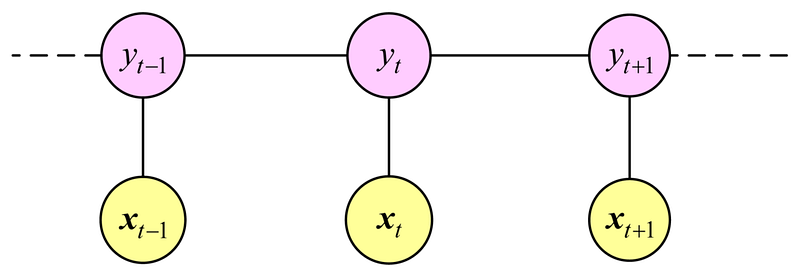Linear Chain Conditional Random Fields

Use Cases: Sequential Data

CRFs are conditional probability distributions that factoirze according to an undirected model. They predict a label for a single sample keeping context from the neighboring samples. CRFs are similar to Hidden Markov Models. CRFs are often used for image segmentation and object recognition, as well as shallow parsing, named entity recognition and gene finding.

# Train for a fixed number of iterations.
session.run(tf.initialize_all_variables())
for i in range(1000):
tf_unary_scores, tf_transition_params, _ = session.run(
[unary_scores, transition_params, train_op])
if i % 100 == 0:
correct_labels = 0
total_labels = 0
for tf_unary_scores_, y_, sequence_length_ in zip(tf_unary_scores, y, sequence_lengths):
# Remove padding from the scores and tag sequence.
tf_unary_scores_ = tf_unary_scores_[:sequence_length_]
y_ = y_[:sequence_length_]

# Compute the highest scoring sequence.
viterbi_sequence, _ = tf.contrib.crf.viterbi_decode(
tf_unary_scores_, tf_transition_params)

# Evaluate word-level accuracy.
correct_labels += np.sum(np.equal(viterbi_sequence, y_))
total_labels += sequence_length_
accuracy = 100.0 * correct_labels / float(total_labels)
print("Accuracy: %.2f%%" % accuracy)

Conclusion

Ever since TensorFlow has been released the community surrounding the project has been adding more packages, examples and cases for using this amazing library. Even at the time of writing this article there are more models and sample code being written. It is amazing to see how much TensorFlow as grown in these past few months. The ease of use and diversity in the package are increasing overtime and don’t seem to be slowing down anytime soon.